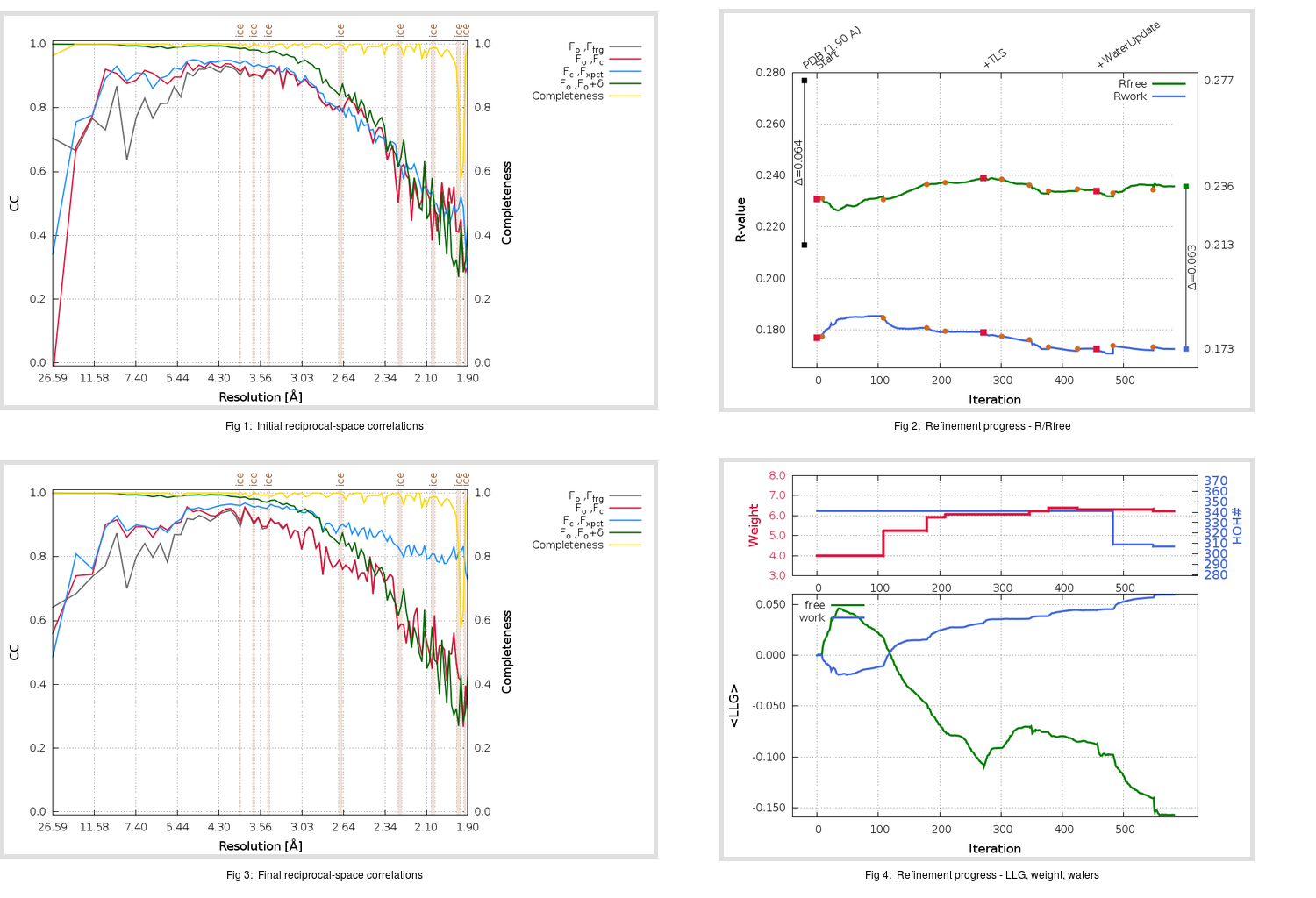Content:

## Deposited

` `
 Date deposited Date data collection Resolution R, Rfree 20200315 20200305 1.90 0.2100 0.2770

Molprobity (CCP4 7.0 version) summary:

```Ramachandran outliers =   0.00 %
favored =  96.03 %
Rotamer outliers      =   1.14 %
C-beta deviations     =     0
Clashscore            =   5.49
RMS(bonds)            =   0.0129
RMS(angles)           =   1.76
MolProbity score      =   1.61
Resolution            =   1.90
R-work                =   0.2100
R-free                =   0.2770
```

```Number of waters      =   341

<B> (all atoms) =   29.86 ( sd =    9.78 ) for       2739 non-hydrogen atoms
<B>   (protein) =   28.89 ( sd =    9.28 ) for       2370 non-hydrogen atoms
<B>     (water) =   35.65 ( sd =   10.62 ) for        341 non-hydrogen atoms
<B>    (others) =   41.90 ( sd =   10.93 ) for         28 non-hydrogen atoms

B min/max       (all non-hydrogen atoms) =   12.51 /   82.90
B min/max   (protein non-hydrogen atoms) =   15.04 /   82.90
B min/max     (water non-hydrogen atoms) =   12.51 /   73.02
B min/max     (other non-hydrogen atoms) =   27.57 /   56.17
```

## BUSTER (re-)refinement

` `

Molprobity (CCP4 7.0 version) summary:

```Ramachandran outliers =   0.33 %
favored =  96.36 %
Rotamer outliers      =   1.89 %
C-beta deviations     =     0
Clashscore            =   3.17
RMS(bonds)            =   0.0117
RMS(angles)           =   1.58
MolProbity score      =   1.56
Resolution            =   1.90
R-work                =   0.1727
R-free                =   0.2359
```

```Number of waters      =   307

<B> (all atoms) =   33.80 ( sd =    8.25 ) for       2705 non-hydrogen atoms
<B>   (protein) =   32.83 ( sd =    7.52 ) for       2370 non-hydrogen atoms
<B>     (water) =   40.30 ( sd =    9.80 ) for        307 non-hydrogen atoms
<B>    (others) =   44.32 ( sd =   12.32 ) for         28 non-hydrogen atoms

B min/max       (all non-hydrogen atoms) =   22.22 /   76.12
B min/max   (protein non-hydrogen atoms) =   22.34 /   68.31
B min/max     (water non-hydrogen atoms) =   22.22 /   76.12
B min/max     (other non-hydrogen atoms) =   31.46 /   59.12
```

Refinement progression:Results:

` `
 File Remark 5RFY_aB_refine.01_03_refine.pdb.gz exact refinement commands are in header 5RFY_aB_refine.01_03_refine.mtz.gz including original deposited data and several re-refinement map coefficients 5RFY_aB_refine.01_03_BUSTER_model.cif.gz including any non-standard compound restraints 5RFY_aB_refine.01_03_BUSTER_refln.cif.gz